RS Aggarwal Class 6 Solutions Chapter 18 - Circles

RS Aggarwal Class 6 Chapter 18 - Circles Solutions Free PDF

RS Aggarwal class 6 solutions chapter 18 circles is provided here. Solve questions of circles from RS Aggarwal for class 6. RS Aggarwal book has all the necessary formulas and theorems which are needed to understand the concepts of circles. To help students solve all the questions from RS Aggarwal we have also provided the RS Aggarwal Class 6 solutions chapter 18 circles.

Exercise 18

Question 1:

Take a point O on your notebook and draw circles of radii 4 cm, 5.3 cm and 6.3 cm, each having the same centre O.

Solution: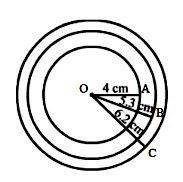This is the required diagram as asked in the question.

Question 2:

Draw a circle with centre C and radius 4.5 cm. Mark points P, Q, R such that P lies in the interior of the circle, Q lies on the circle, and R lies in the exterior of the circle.

Solution: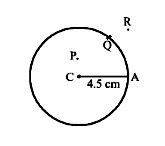This is the required diagram as asked in the question.

Question 3:

Draw a circle with centre O and radius 4 cm. Draw a chord AB of the circle. Indicate by marking points X and Y, the minor arc AXB and the major arc AYB of the circle.

Solution: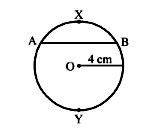This is the required diagram as asked in the question.

Question 4:

Which of the following statements are true and which are false?

(i) Each radius of a circle is also a chord of the circle.

(ii) Each diameter of a circle is also a chord of the circle.

(iii) The centre of a circle bisects each chord of the circle.

(iv) A secant of a circle is a segment having its end points on the circle.

(v) A chord of a circle is a segment having its end points on the circle.

Solution:

(i) False

Diameter of a circle is a chord of the circle, not radius.

(ii) True

It is the longest chord of the circle.

(iii) False

A perpendicular drawn from the centre of the circle to the chord, bisects the chord.

(iv) False

It is a line passing through the circle that intersects the circle at two points.

(v) True

Question 5:

Draw a circle with centre O and radius 3.7 cm. Draw a sector having the angle 72o.

Solution: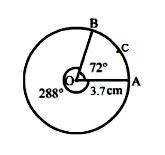Therefore, the required arc is arc OACB.

Question 6:

Fill in the blanks by using <, >, = or $leq$..

(i) OP _____ OQ, where O is the centre of the circle, P lies on the circle and Q is in the interior of the circle.

(ii) OP _____ OR, where O is the centre of the circle, P lies on the circle and R lies in the exterior of the circle.

(iii) Major arc _______ minor arc of the circle.

(iv) Major arc _______ semi circumference of the circle.

Solution:

(i) >

(ii) <

(iii) >

(iv) >

This is because the major arc covers more than half of the circumference of the circle.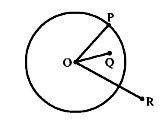Question 7:

Fill in the blanks:

(i) A diameter of a circle is a chord that _________ the centre.

(ii) A radius of a circle is a line segment with one end point ________ and the other end point __________ .

(iii) If we join any two points of a circle by a line segment, we obtain a _________ of a circle.

(iv) Any part of a circle is called an ________ of an circle.

(v) The figure bounded by an arc and the two radii joining the end points of the arc with the centre is called a __________ of the circle.

Solution:

(i) passes through

(ii) on the circle, at the centre of the circle

(iii) chord

(iv) arc

(v) sector

Practise This Question

Electricity is very essential for day to day activities.